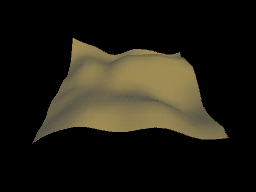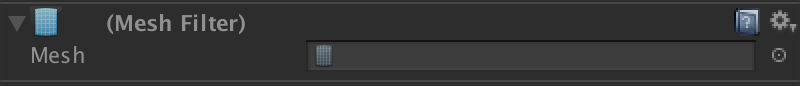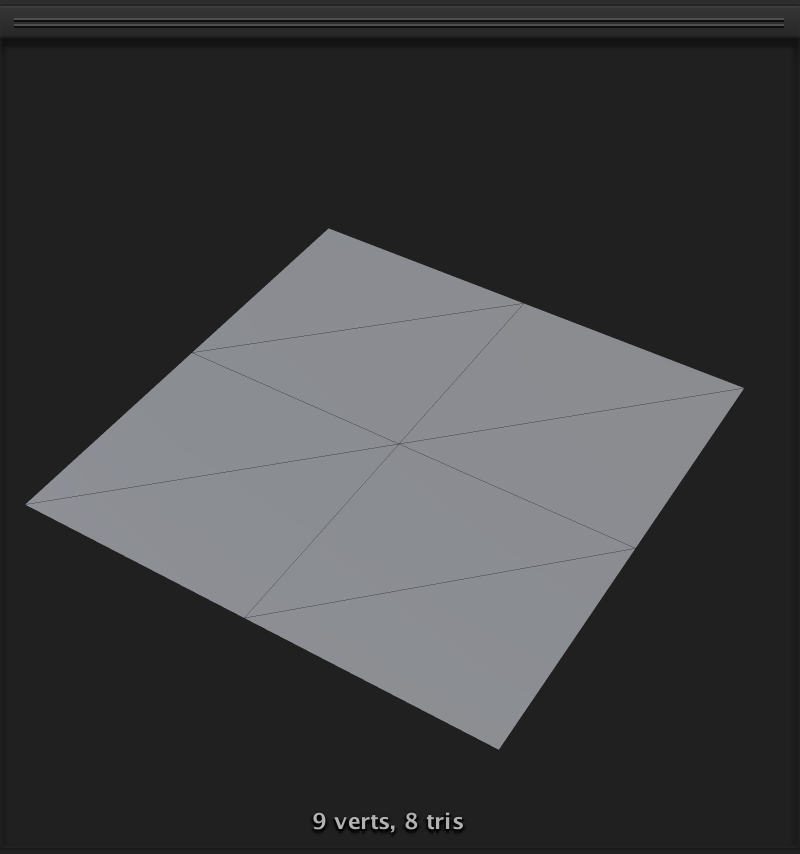# Mesh Generation in Unity

## Mesh Generation in UnityWriting scripts for dynamic mesh generation can be annoying and full of gotchas, but very powerful when successful! It took me a while to feel comfortable with it, so I thought I’d compile some of the tricks I picked up into a tutorial. None of these ideas are specific to volumetric or multi-view displays, but the results tend to be some of the best-looking content for those mediums.

Experience Level: Intermediate
This tutorial assumes you know a few basics of coding in Unity, such as what a Mesh Filter and Mesh Renderer are, how to define a class, and how to use for-loops.

This will be a 3 part series, so don’t miss parts 2 and 3.

## Part 1

Overview:

• Create a `MeshData` class
• Generate a grid of quads

In a blank scene in Unity, create an empty GameObject, and name it “Mesh Generator” (or whatever you’d like). Add a Mesh Renderer and Mesh Filter component to it. Also add a new component and call it MeshGenerator.

Open up the MeshGenerator script. The first thing we want to do is define a custom class called MeshData. This is where we’ll store the lists of verts, tris, and uvs, so we can more readily manipulate them. Also included is a `ClearAll()` function for convenience, if or when we want to start building the mesh from scratch again.

``````    public class MeshData
{
public List<Vector3> verts = new List<Vector3>();
public List<int> tris = new List<int>();
public List<Vector2> uv = new List<Vector2>();
public void ClearAll()
{
verts.Clear();
tris.Clear();
uv.Clear();
}
}
``````

It might seem redundant to do this: doesn’t the built-in `Mesh` class already have these properties? Yes, it does, but it is bad and/or impossible to edit them in a progressive manner like we’ll be doing. Instead we keep adding to our `MeshData` lists, and when we’re all done, we set the `Mesh` properties from those lists. I assure you this is a regular thing that people do all the time.

We can create our `MeshGenerator` class properties now. Add a field for the instance of our `MeshData` and initialize it. In our `Awake()` function, we can set the reference for the `meshFilter` and create a new mesh for it as well.

``````   public class MeshData
{
public List<Vector3> verts = new List<Vector3>();
public List<int> tris = new List<int>();
public List<Vector2> uv = new List<Vector2>();
public void ClearAll()
{
verts.Clear();
tris.Clear();
uv.Clear();
}
}

MeshData meshData = new MeshData();
MeshFilter meshFilter;

void Awake()
{
meshFilter = GetComponent<MeshFilter>();
meshFilter.mesh = new Mesh();
}
``````

Now we’re ready to make some meshes baby!

Let’s add two public fields up near the others: width and length

``````    MeshData meshData = new MeshData();
MeshFilter meshFilter;

public int width = 3;
public int length = 3;
``````

These define how long and how wide our mesh is. By default I set them to 3, but you can adjust them in the inspector window.

Make a new function called `GenerateMesh()` like so:

``````    void GenerateMesh()
{
meshData.ClearAll();
for (int z = 0; z < length; z++)
{
for (int x = 0; x < width; x++)
{

}
}
}
``````

We start by clearing the meshData, for the hypothetical situation that we’ve already created a Mesh and want to start over. Then we set up a nested for-loop. This will give us our coordinates as we march through and create our verts and tris. You can imagine if we were to write `print(x + ", " + z)` in the middle of the loop, the output would be:
`0, 0`
`1, 0`
`2, 0`
`0, 1`
`1, 1`
`2, 1`
`0, 2`
`1, 2`
`2, 2`

It’s a two-dimensional set of coordinates, but we are using `z` instead of `y` because it proceeds forward in our example, not up. Now let’s add a vert for each of these coordinates we’ll be hitting, like so:

``````        for (int z = 0; z < length; z++)
{
for (int x = 0; x < width; x++)
{
}
}
``````

Good! Now we have a 3x3 array of vertices. Now for the fun part: stitching these into triangles.

If you’ve never dealt with mesh data before, it might seem confusing that the tris are listed as a 1-dimensional array of integers. But it’s actually very clever! You can assume that since a triangle is always made up of 3 points, the ints in the array represent the index of the verts that make up each triangle, three at a time. So, for example, this set of tris: `[0, 1, 2, 2, 3, 0]` instructs the mesh interpreter to make one triangle out of vertices 0, 1, and 2, and a second triangle out of verts 2, 3, and 0.

We want to stitch two triangles for every vertex, giving us a quad. In this diagram, the red diamonds are vertices, and the arrows represent the steps in drawing our triangles.For this set of triangles, we’d be adding [0, 3, 4] and [4, 1, 0] to the tris list. But knowing the hard numeric values is not very useful to us! We need to know the indices of these verts in a dynamic sense, so it can be iteratively built for us.

Let’s add some conditionals. Think about the triangles we are drawing: they wouldn’t be possible until we reach the 4th vertex in the loop. So as long as `x == 0` or `z == 0` (i.e. we’re still on the first row or first column), we shouldn’t be stitching triangles yet.

``````                if (x != 0 && z != 0)
{

}
``````

Since we’re using vertex 4 in this diagram as a starting point, what would that value be considered dynamically? It’s `x + z * width`. How do we know that? Well, `z` iterates once `x` is finished with its loop, so for every `z` incrementation, the index is greater by that many times `width`. Think of it like this: to move up or down on the imaginary “y” axis of this 1D array, we add or subtract by whole widths at a time.

Let’s call that `v4`:
`int v4 = x + z * width;`

So what is v3?
`v4 - 1` is the obvious answer, and thankfully the correct one.

What about v1 and v0? Here’s where it helps to really internalize this concept of traversing a 1 dimensional array which represents 2d coordinates. To move up and down on the vertical axis, we’re simply adding or subtracting by `width`.

So vertex 1 is
`v4 - width`
and vertex 0 is
`v3 - width`.

Now let’s add those triangles to the list in the order they are shown in the diagram!

``````                if (x != 0 && z != 0)
{
int v4 = x + z * width;
int v3 = v4 - 1;
int v1 = v4 - width;
int v0 = v3 - width;
meshData.tris.AddRange(new []{v0, v3, v4}); //tri 1
meshData.tris.AddRange(new []{v4, v1, v0}); //tri 2
}
``````

Finally, outside of your nested for-loop, once everything is done, remember to set the actual verts and tris of the real mesh.

``````        meshFilter.sharedMesh.SetVertices(meshData.verts);
meshFilter.sharedMesh.SetTriangles(meshData.tris, 0);
``````

I set them on `sharedMesh` because that prevents us from instantiating an entirely new mesh if we are doing this in Edit mode.

Finally finally, add `GenerateMesh()` to your awake function:

``````    void Awake()
{
meshFilter = GetComponent<MeshFilter>();
meshFilter.mesh = new Mesh();
GenerateMesh();
}
``````

And press play!

You should see something remarkably underwhelming, like so:But if you check the mesh in the inspector, by double clicking on the nameless mesh we createdYou will see in the preview at the bottom of the inspector (you may have to drag it up from the bottom to see it) that we have created a quad with tris in the order we want them!This is super powerful! Lets change the width and length to 16 and see what we get:This still looks pretty boring, but believe me…it’s good. We can use this setup to do all sorts of things:

• terrain
• waves

that’s it but those are very good things.

Thanks for reading and if you have any questions (or, likely, corrections) message me or reply here!

In part 2 we’ll cover:

• Noise
1 Like

This is great material. Will you be continuing?